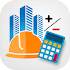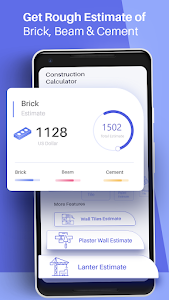# Construction Estimator App v1.3

Free

### Construction Estimator App / Screenshots### Construction Estimator App / Description

Construction estimator app is the simple yet best app to calculate estimated price of building material required for construction. You can get a construction material quantity by using this app to calculate material required for Building or House Construction. This is a specially designed for calculate civil quantity. This is a very helpful app for Builders as it is very easy to use as building cost estimator. You just have to fill a Building dimensions form and this app will provide you with a cost summary. Building Material Estimate App uses optimistic formulas to solve the construction calculation through this simple construction mobile app. This construction price calculator helps you to calculate the cost of materials used for construction in a very quick time.

Estimation of material cost is the most challenging work as we don’t know what will be the cost of construction. This Construction app can be used as a Brick calculator as it helps you to calculate amount of bricks as well as to calculate cost for bricks required for a building. Furthermore This Material Estimator App can be used as a Tiles Calculator and Paint Estimator. We might find it difficult to find the overall cost estimation of a building construction. Our Construction Calculator have a lots of Cost calculation features to give you an estimate of Total cost of a building in a single app.

Our main material and cost calculation features of this app includes

-Bricks Estimation: Bricks are the basic and most important construction material in the construction of buildings even if you want to construct a house. Estimator app asks for proper room dimensions and Cost of Brick to calculate total number of bricks and Total Bricks cost in Rupees.

-Tile Estimation: Tiles calculator asks for Wall and floor Dimensions and price of one tile to calculate the amount of tiles require. Wall tile estimator will give you total wall tiles required along with the Total cost of tiles in a room. Floor tile estimator gives total floor tiles required.

-Paint Estimation: Paint estimator calculates the amount of paint buckets required to paint a room. Paint calculator also asks for Door dimensions and window dimensions for accurate calculation of paint Buckets.

-Slab Estimation: Our app asks for slab dimensions and dimensions of required steel for construction to calculate the no of bars and steel cost.

-Beam Estimation: This app asks for Beam dimensions and dimensions of required total no of bars required to calculate bars and beam cost.

-Plaster Estimation: Wall Plaster Estimator can calculate cement bags. Ceiling Plaster Estimator can calculate amount of cement bags required for construction of a room.